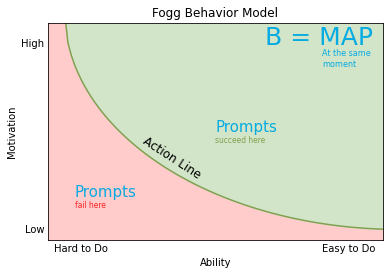4 minutes

In this post, I would like to explain the Fogg Behavior Model, created by Brian Jeffrey Fogg (B. J. Fogg).

According to B. J. Fogg's research, Behavior ($B$) happens at the intersection of three things: our Motivation ($M$), our Ability ($A$), and the Prompt ($P$) to the behavior.

$B = MAP$

We can visualize the relationship between motivation, ability and the prompt in a two-dimensional graphic.

I plotted the graphic below using Python and the NumPy and Matplotlib packages. I kept the code for anyone who is interested in how to do it.

In :
import matplotlib.pyplot as plt
import numpy as np

text = { # text dict for easier translation
'ability':'Ability',
'motivation':'Motivation',
'title':'Fogg Behavior Model',
'bmap':'B = MAP',
'bmap_sub':'At the same\nmoment',
'ability_axis':('Hard to Do', 'Easy to Do'),
'motivation_axis':('High', 'Low'),
'action_line':'Action Line',
'prompts':'Prompts',
'prompts_succeed':'succeed here',
'prompts_fail':'fail here',
}

blue_color = '#06abe1'
green_color = '#81a050'
light_green_color = '#d2e5c9'
red_color = '#ff2222'
light_red_color = '#ffcbcb'

# Data for plotting
x = np.arange(0.0, 1.1, 0.01)
# y = 1.05 - sqrt(1 - (x - 1.05) ** 2)
y = 1.05 - np.sqrt([np.max((xi, 0)) for xi in 1 - (x - 1.05) ** 2])

fig, ax = plt.subplots()
ax.plot(x, y, color=green_color)
ax.set(xlabel=text['ability'],
ylabel=text['motivation'],
title=text['title'])
ax.tick_params(axis='both', which='both',length=0)
ax.fill_between(x, y, color=light_red_color)
ax.fill_between(x, y, max(y), color=light_green_color)

ax.text(0.65, 0.9, text['bmap'], size=25, color=blue_color)
ax.text(0.82, 0.80, text['bmap_sub'], size=8, color=blue_color)

prompts_size=15
ax.text(0.5, 0.5, text['prompts'], size=prompts_size, color=blue_color)
ax.text(0.5, 0.45, text['prompts_succeed'], size=prompts_size*0.5, color=green_color)
ax.text(0.08, 0.2, text['prompts'], size=prompts_size, color=blue_color)
ax.text(0.08, 0.15, text['prompts_fail'], size=prompts_size*0.5, color=red_color)

# set x axis ticks names
xticks = [''] * (len(x)-10)
xticks, xticks[-10] = text['ability_axis']
plt.xticks(x, xticks)
# set y axis ticks names
yticks = [''] * (len(y)-10)
yticks, yticks[-1] = text['motivation_axis']
plt.yticks(y, yticks)

# set axis' limits
plt.xlim([0.0, 1.0])
plt.ylim([0.0, 1.0])

tp = np.array((0.28, 0.45))
trans_angle = plt.gca().transData.transform_angles(np.array((315,)),
tp.reshape((1, 2)))
plt.text(tp, tp, text['action_line'], fontsize=12,
rotation=trans_angle, rotation_mode='anchor')
plt.show()• Image based in the Fogg Behavior Model (as presented in the book), also inspired by this one found in Google Images.

Looking at the graphic we can see that if there is a prompt for a behavior, but it is something too hard to do or if the motivation is low, the behavior will fail.

On the contrary, if the behavior is easy to do and we have the motivation when the prompt surges the behavior will be a success!

Thinking in the process of building new habits, we could use this model as a guide on how to develop a new one. After choosing the right behavior, make sure you have the right motivation for it and make it really easy to do it! In other words, you should make it as simple and small so that there is no chance you will not do it. In this way, each time you are successful with your behavior you are taking a step in making it a new habit.

A similar conclusion can be thought in the process of stopping a bad habit, make it really hard to do, so that even when the prompt arises you will be less likely to do it.

This is the suggested template by B. J. Fogg to create a new behavior:

After I ..., I will ... . Then, I celebrate!

The celebration is an important step for you to connect the behavior with something positive that should be repeated.

If you think the behavior you tried to create is not working, try this troubleshoot method:

1. Check prompt
2. Check ability
3. Check motivation

And keep trying out and learning more about yourself, one small step at a time.